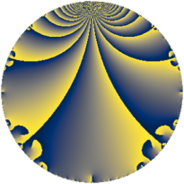# Properties

 Label 3675.2.a.jLevel $3675$ Weight $2$ Character orbit 3675.a Self dual yes Analytic conductor $29.345$ Analytic rank $0$ Dimension $1$ CM no Inner twists $1$

# Related objects

## Newspace parameters

 Level: $$N$$ $$=$$ $$3675 = 3 \cdot 5^{2} \cdot 7^{2}$$ Weight: $$k$$ $$=$$ $$2$$ Character orbit: $$[\chi]$$ $$=$$ 3675.a (trivial)

## Newform invariants

 Self dual: yes Analytic conductor: $$29.3450227428$$ Analytic rank: $$0$$ Dimension: $$1$$ Coefficient field: $$\mathbb{Q}$$ Coefficient ring: $$\mathbb{Z}$$ Coefficient ring index: $$1$$ Twist minimal: no (minimal twist has level 15) Fricke sign: $$-1$$ Sato-Tate group: $\mathrm{SU}(2)$

## $q$-expansion

 $$f(q)$$ $$=$$ $$q + q^{2} - q^{3} - q^{4} - q^{6} - 3q^{8} + q^{9} + O(q^{10})$$ $$q + q^{2} - q^{3} - q^{4} - q^{6} - 3q^{8} + q^{9} - 4q^{11} + q^{12} - 2q^{13} - q^{16} + 2q^{17} + q^{18} - 4q^{19} - 4q^{22} + 3q^{24} - 2q^{26} - q^{27} - 2q^{29} + 5q^{32} + 4q^{33} + 2q^{34} - q^{36} + 10q^{37} - 4q^{38} + 2q^{39} - 10q^{41} - 4q^{43} + 4q^{44} + 8q^{47} + q^{48} - 2q^{51} + 2q^{52} + 10q^{53} - q^{54} + 4q^{57} - 2q^{58} + 4q^{59} + 2q^{61} + 7q^{64} + 4q^{66} - 12q^{67} - 2q^{68} - 8q^{71} - 3q^{72} + 10q^{73} + 10q^{74} + 4q^{76} + 2q^{78} + q^{81} - 10q^{82} + 12q^{83} - 4q^{86} + 2q^{87} + 12q^{88} + 6q^{89} + 8q^{94} - 5q^{96} + 2q^{97} - 4q^{99} + O(q^{100})$$

## Embeddings

For each embedding $$\iota_m$$ of the coefficient field, the values $$\iota_m(a_n)$$ are shown below.

For more information on an embedded modular form you can click on its label.

Label $$\iota_m(\nu)$$ $$a_{2}$$ $$a_{3}$$ $$a_{4}$$ $$a_{5}$$ $$a_{6}$$ $$a_{7}$$ $$a_{8}$$ $$a_{9}$$ $$a_{10}$$
1.1
 0
1.00000 −1.00000 −1.00000 0 −1.00000 0 −3.00000 1.00000 0
 $$n$$: e.g. 2-40 or 990-1000 Significant digits: Format: Complex embeddings Normalized embeddings Satake parameters Satake angles

## Atkin-Lehner signs

$$p$$ Sign
$$3$$ $$1$$
$$5$$ $$1$$
$$7$$ $$-1$$

## Inner twists

This newform does not admit any (nontrivial) inner twists.

## Twists

By twisting character orbit
Char Parity Ord Mult Type Twist Min Dim
1.a even 1 1 trivial 3675.2.a.j 1
5.b even 2 1 735.2.a.c 1
7.b odd 2 1 75.2.a.b 1
15.d odd 2 1 2205.2.a.i 1
21.c even 2 1 225.2.a.b 1
28.d even 2 1 1200.2.a.e 1
35.c odd 2 1 15.2.a.a 1
35.f even 4 2 75.2.b.b 2
35.i odd 6 2 735.2.i.e 2
35.j even 6 2 735.2.i.d 2
56.e even 2 1 4800.2.a.bz 1
56.h odd 2 1 4800.2.a.t 1
77.b even 2 1 9075.2.a.g 1
84.h odd 2 1 3600.2.a.u 1
105.g even 2 1 45.2.a.a 1
105.k odd 4 2 225.2.b.b 2
140.c even 2 1 240.2.a.d 1
140.j odd 4 2 1200.2.f.h 2
280.c odd 2 1 960.2.a.l 1
280.n even 2 1 960.2.a.a 1
280.s even 4 2 4800.2.f.bf 2
280.y odd 4 2 4800.2.f.c 2
315.z even 6 2 405.2.e.c 2
315.bg odd 6 2 405.2.e.f 2
385.h even 2 1 1815.2.a.d 1
420.o odd 2 1 720.2.a.c 1
420.w even 4 2 3600.2.f.e 2
455.h odd 2 1 2535.2.a.j 1
560.be even 4 2 3840.2.k.r 2
560.bf odd 4 2 3840.2.k.m 2
595.b odd 2 1 4335.2.a.c 1
665.g even 2 1 5415.2.a.j 1
805.d even 2 1 7935.2.a.d 1
840.b odd 2 1 2880.2.a.bc 1
840.u even 2 1 2880.2.a.y 1
1155.e odd 2 1 5445.2.a.c 1
1365.g even 2 1 7605.2.a.g 1

By twisted newform orbit
Twist Min Dim Char Parity Ord Mult Type
15.2.a.a 1 35.c odd 2 1
45.2.a.a 1 105.g even 2 1
75.2.a.b 1 7.b odd 2 1
75.2.b.b 2 35.f even 4 2
225.2.a.b 1 21.c even 2 1
225.2.b.b 2 105.k odd 4 2
240.2.a.d 1 140.c even 2 1
405.2.e.c 2 315.z even 6 2
405.2.e.f 2 315.bg odd 6 2
720.2.a.c 1 420.o odd 2 1
735.2.a.c 1 5.b even 2 1
735.2.i.d 2 35.j even 6 2
735.2.i.e 2 35.i odd 6 2
960.2.a.a 1 280.n even 2 1
960.2.a.l 1 280.c odd 2 1
1200.2.a.e 1 28.d even 2 1
1200.2.f.h 2 140.j odd 4 2
1815.2.a.d 1 385.h even 2 1
2205.2.a.i 1 15.d odd 2 1
2535.2.a.j 1 455.h odd 2 1
2880.2.a.y 1 840.u even 2 1
2880.2.a.bc 1 840.b odd 2 1
3600.2.a.u 1 84.h odd 2 1
3600.2.f.e 2 420.w even 4 2
3675.2.a.j 1 1.a even 1 1 trivial
3840.2.k.m 2 560.bf odd 4 2
3840.2.k.r 2 560.be even 4 2
4335.2.a.c 1 595.b odd 2 1
4800.2.a.t 1 56.h odd 2 1
4800.2.a.bz 1 56.e even 2 1
4800.2.f.c 2 280.y odd 4 2
4800.2.f.bf 2 280.s even 4 2
5415.2.a.j 1 665.g even 2 1
5445.2.a.c 1 1155.e odd 2 1
7605.2.a.g 1 1365.g even 2 1
7935.2.a.d 1 805.d even 2 1
9075.2.a.g 1 77.b even 2 1

## Hecke kernels

This newform subspace can be constructed as the intersection of the kernels of the following linear operators acting on $$S_{2}^{\mathrm{new}}(\Gamma_0(3675))$$:

 $$T_{2} - 1$$ $$T_{11} + 4$$ $$T_{13} + 2$$

## Hecke characteristic polynomials

$p$ $F_p(T)$
$2$ $$-1 + T$$
$3$ $$1 + T$$
$5$ $$T$$
$7$ $$T$$
$11$ $$4 + T$$
$13$ $$2 + T$$
$17$ $$-2 + T$$
$19$ $$4 + T$$
$23$ $$T$$
$29$ $$2 + T$$
$31$ $$T$$
$37$ $$-10 + T$$
$41$ $$10 + T$$
$43$ $$4 + T$$
$47$ $$-8 + T$$
$53$ $$-10 + T$$
$59$ $$-4 + T$$
$61$ $$-2 + T$$
$67$ $$12 + T$$
$71$ $$8 + T$$
$73$ $$-10 + T$$
$79$ $$T$$
$83$ $$-12 + T$$
$89$ $$-6 + T$$
$97$ $$-2 + T$$